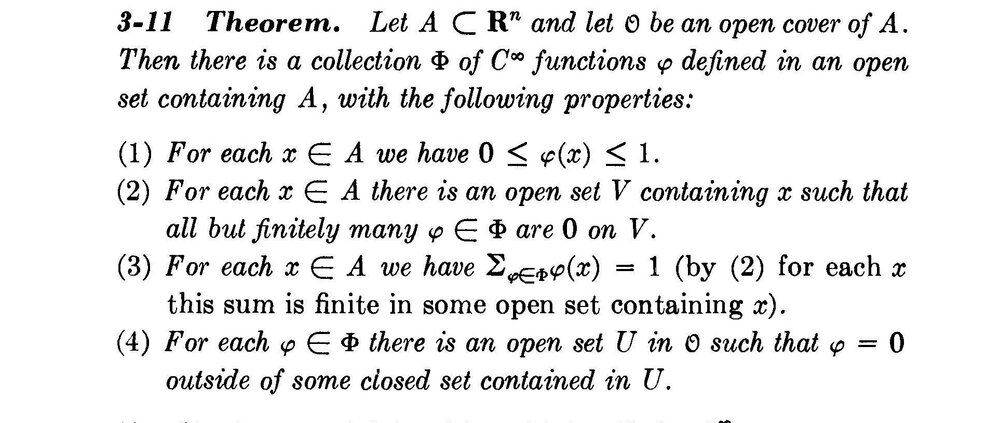# Problems with understanding the role of the partition of unity

• I
Santiago24
I'm reading "Calculus on manifolds" by Spivak and i can't understand the role that the partition of unity play and why this properties are important , Spivak say:What is the purpose of the partition of unity? if someone can give me examples, bibliography or clear my doubt i'll appreciate it.

•aclaret

Homework Helper
Gold Member
We can use them to put together smooth objects, such as functions, that are only defined on parts of a manifold (patches) to make a smooth global object.

Here is an example of creating a partition of unity that gives us two nonzero, smooth functions defined on the unit circle ##S^1##, that add to 1 everywhere.

The second para of this wolfram page gives an example of how we can use the general partition of unity theorem (of which the theorem you quote above is a special case, using the manifold ##\mathbb R^n##) to prove that any manifold can have smooth vector fields on it that are not everywhere zero.

This lists other applications. I find the signal processing filter particularly interesting.

•Santiago24 and fresh_42
Santiago24
We can use them to put together smooth objects, such as functions, that are only defined on parts of a manifold (patches) to make a smooth global object.

Here is an example of creating a partition of unity that gives us two nonzero, smooth functions defined on the unit circle ##S^1##, that add to 1 everywhere.

The second para of this wolfram page gives an example of how we can use the general partition of unity theorem (of which the theorem you quote above is a special case, using the manifold ##\mathbb R^n##) to prove that any manifold can have smooth vector fields on it that are not everywhere zero.

This lists other applications. I find the signal processing filter particularly interesting.